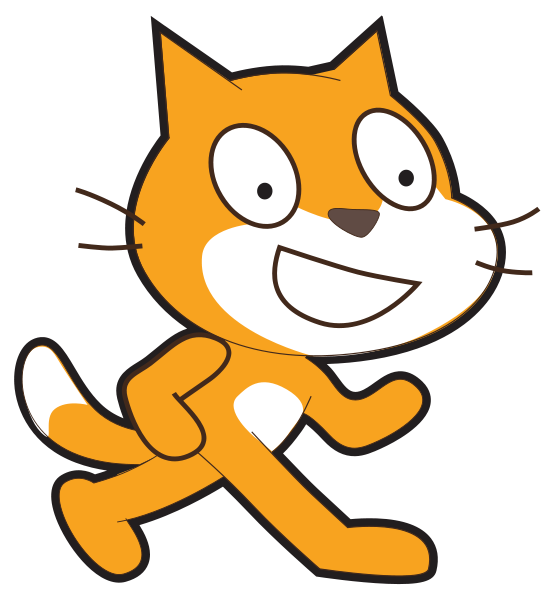# 12 digit product codes: Weighted sum of digits (entered one at a time)

Challenge Level: Growing experience

Learning outcomesStudents will be able to:

## Requirement:

Write a program that asks the user to enter a 12 digit product code, entering each digit one at a time. Then adds up all the digits, every second one multiplied by 3 (1st, 3rd, and so on), and shows the total as the output.

## Testing examples:

Your program should display the outputs shown in this table for the given inputs provided:

Input Output
8
3
8
3
1
0
0
2
2
5
2
4
Total: 80

## Languages

Scratch#### What it should look like

Click on the green flag, enter the inputs provided in the “testing examples” to see the expected output of your program.

Recommended blocks
when green flag clicked
ask (join (join [Enter digit ] (counter)) [ of the product code:]) and wait

ask (join (join [Enter digit ] (counter)) [ of the product code:]) and wait
repeat (6)
end

if <((total) mod (10)) = > then
else
end
say [The sum is a multiple of 10]

say [The sum is NOT a multiple of 10]
set [total 1 v] to 

set [total 2 v] to 

set [counter v] to 

change [counter v] by (1)

change [total 1 v] by (answer)

change [counter v] by (1)

change [total 2 v] by (answer)

set [total v] to (((total 1) * (3)) + (total 2))
Hints
• You need to add up 1st, 3rd, 5th and so on digits and store the result in a variable called total 1. Then add up 2nd, 4th, 6th and so on digits and store the result in variable called total 2. Store the sum of total 1 multiplied by 3 and total 2 in a variable called total. The output displays if this total is a multiple of 10 or not (i.e. if “total” mod 10 is equal to 0).